Multiple Choice
Identify the choice that best completes the statement or answers the question.

1.

How many feet does the arrow on this scale indicate if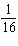:1?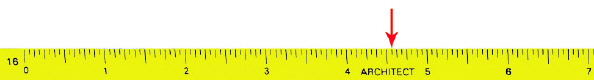a. 73 c. 49 b. 4.6 d. 46

2.

How many feet does the arrow on this scale indicate if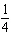:1?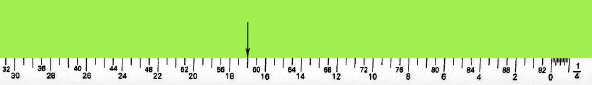a. 16.5 c. 56.75 b. 59 d. 17

3.

How many feet does the arrow on this scale indicate if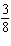:1?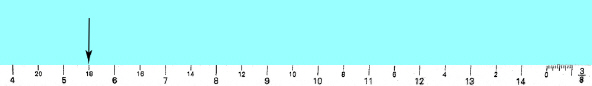a. 18 c. 6.5 b. 5.5 d. 17

4.

How many feet does the arrow on this scale indicate if:1?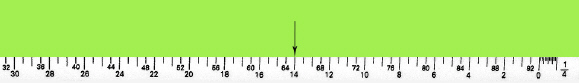a. 65 c. 13.5 b. 14 d. 64.25

5.

How many feet does the arrow on this scale indicate if:1?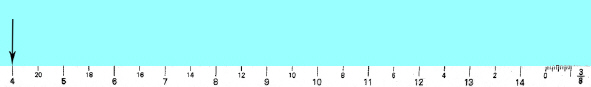a. 4 c. 22 b. 20.5 d. 21

6.

How many feet does the arrow on this scale indicate if:1?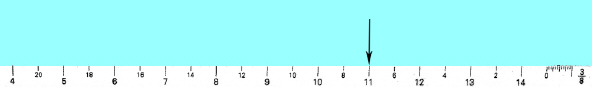a. 11 c. 7 b. 9 d. 10

7.

How many feet does the arrow indicate on this scale if:1?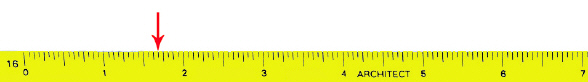a. 18 c. 5 b. 27 d. 21

8.

How many feet does the arrow on this scale indicate if:1?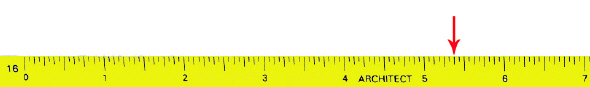a. 56 c. 83 b. 5.3 d. 8.5

9.

How many feet does the arrow on this scale represent if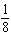:1?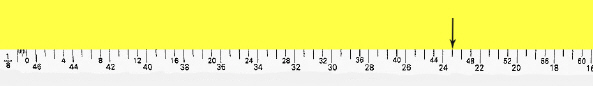a. 24.5 c. 44.5 b. 23 d. 46

10.

How many feet does the arrow on this scale indicate if:1?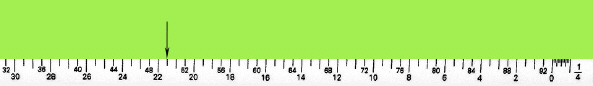a. 50 c. 21.5 b. 23 d. 23

11.

How many feet does the arrow on this scale indicate if:1?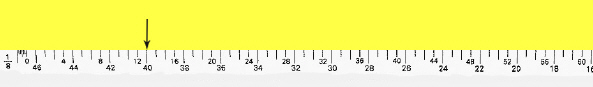a. 13 c. 12.25 b. 40 d. 11

12.

How many feet does the arrow on this scale indicate if:1?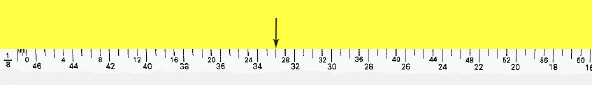a. 27 c. 29 b. 33 d. 36